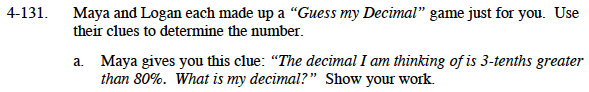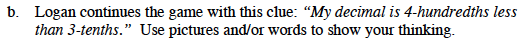### Home > MC1 > Chapter 4 > Lesson 4.3.5 > Problem4-131

4-131.
1. . Maya and Logan each made up a "Guess my Decimal" game just for you. Use their clues to determine the number. Homework Help ✎

1. Maya gives you this clue: "The decimal I am thinking of is 3-tenths greater than 80%. What is my decimal? " Show your work.

2. Logan continues the game with this clue: "My decimal is 4-hundredths less than 3-tenths." Use pictures and/or words to show your thinking.$\text{If we know that 80\% is }\frac{80}{100}, \text{ which is equal to } \frac{8}{10},\text{ what number is }\frac{3}{10}\text{ more than that?}$

$\text{Maya's decimal is 1.1. \ The fraction of this decimal is } \frac{11}{10}\text{ or }\frac{110}{100}.$$\text{Can you convert }\frac{3}{10}\text{ to hundredths? This will make it easier to subtract } \frac{3}{100}\text{ in order to find Logan's fraction. }$

$\text{If the fraction of Logan's decimal is }\frac{27}{100},\text{ can you find the decimal?}$